GRE Data Analysis | Methods for Presenting Data

• Last Updated : 01 Jul, 2019

Data Interpretation Question in GRE will require the knowledge of understanding Data Representation methods. It is very Important to understand data correctly and apply the same to solve the problem.

Important Terms to know –

• Variable:
A characteristics which varies with respect to given categories.
There are two types of variables.
1. Quantitative or numerical – e.g. age of individuals or quantity of televisions per household.
2. Categorical or non-numerical – e.g. eye color of individuals or blood group of observed individuals.
• Frequency OR Count:
Number of appearances of a particular category or value in the table.
• Frequency Distribution:
Table or graph representing categories along with their corresponding frequencies.
• Relative Frequency:• Relative Frequency Distribution:
Table or graph representing relative frequencies of the categories.

There are various methods to represent data which are mentioned below:

1. Tables
2. Bar Graphs
3. Segmented Bar Graphs
4. Histograms
5. Circle Graphs
6. Scatter Plots
7. Line Graphs

Some of these explained as following below.

1. Tables:

• In tables, intersection of rows and column defines particular data.
• Rows determine relation between horizontal elements and columns determine relation between vertical elements.
• Data can be in numeric form or percentage form.

Representation of Data in Table form:

Book CategoriesBooks available at a section
Biographies and memoirs60
Children’s books81
Comics56
Engineering54
Fiction and literature50
History41
Religion and Spirituality58
Total400

From above table, we can infer that there are total 400 books in a particular section and from that 60 books are of Biographies Category.

Similarly, number assigned under “Books available at a Section” represents corresponding “Book Category”.
This same table can be presented as percentage as well. Instead of numbers, percentage of total quantity is given and we have to derive in number separately if required. It is not necessary that the table representation have two columns. In case we want to represent data of student name, student ID, percentage achieved, marks received in each subjects, etc. then the number of columns would be greater than two.

2. Bar Graphs:
In Bar Graphs, data is represented in XY plane to determine the relation between two or more quantities.

Bar Graph Representation: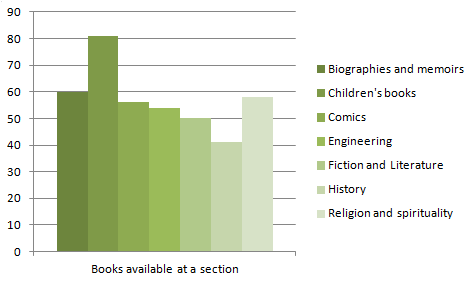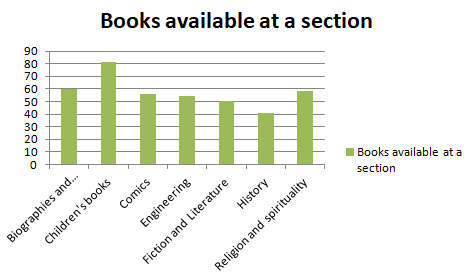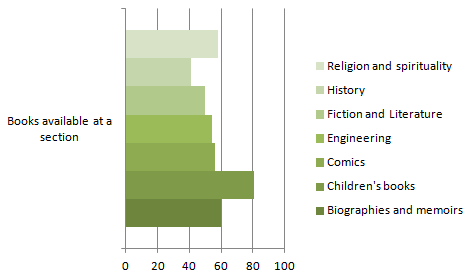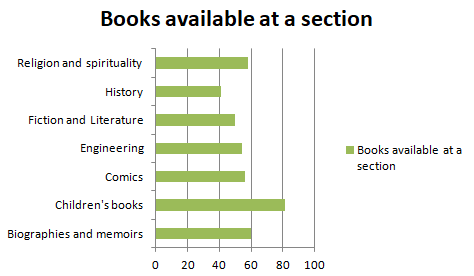Here, two variables – Category of book and number of books available at a particular section – are correlated by XY plane.
Bar graph can be represented horizontally or vertically. It is also possible to correlate three variables and represent it in a bar graph form.

Suppose, in this same graph, we want to categorize books published before 2000 and after 2000, it is possible. In that case, the bars will be divided into two parts and as per the assignment of the years, it will indicate the information. It is called Segmented Bar Graphs. It is also represented by two joint bars for each category instead of bisecting the bars.

3. Circle Graphs:
Circle Graphs are represented by segments of a circle. It is also frequently refereed as Pie Charts.

Circle Graph Representation: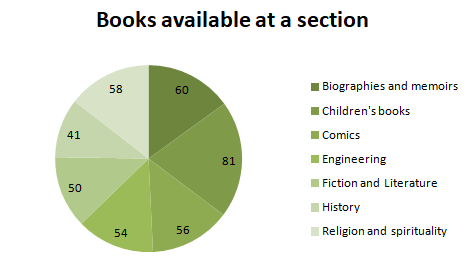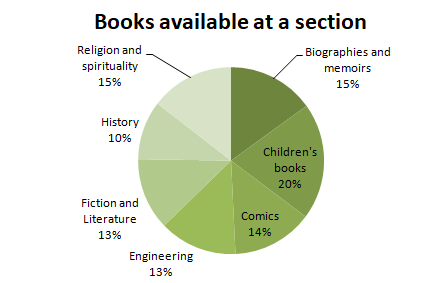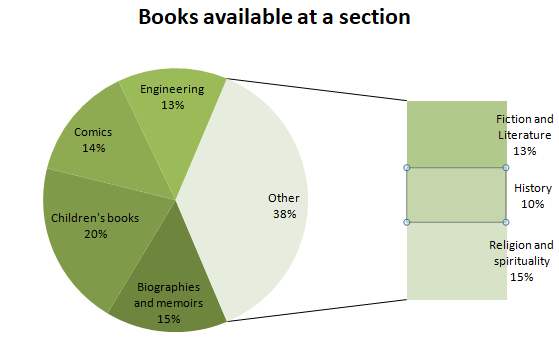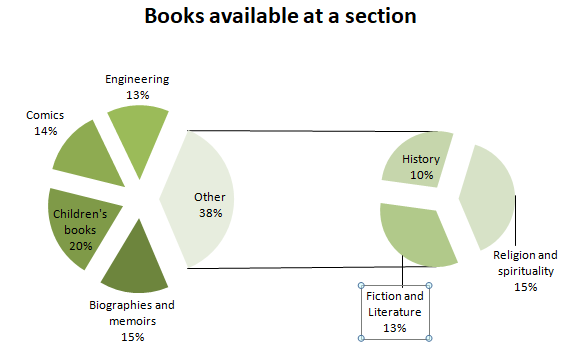Scatter Plots and Line Graphs:
Line Graphs are represented by joining points of intersection and drawing a simple line. The flow of line represents the relation between both the axis categories. Scatter Plots are represented by points around a reference line. It is also represented on XY plane and defines the relation between X and Y segments but a third variable cannot be represented in this type of graph.

Line Graph Representation: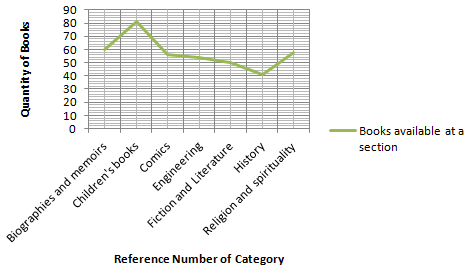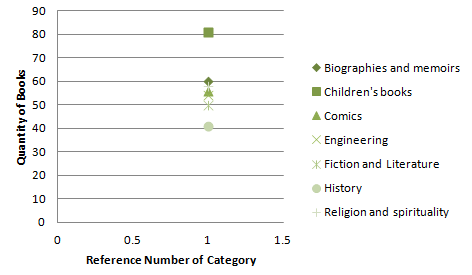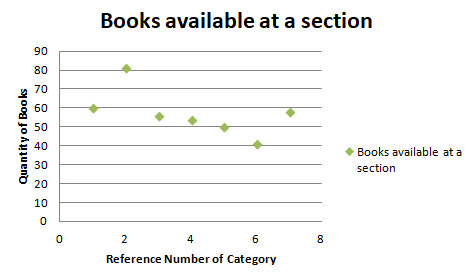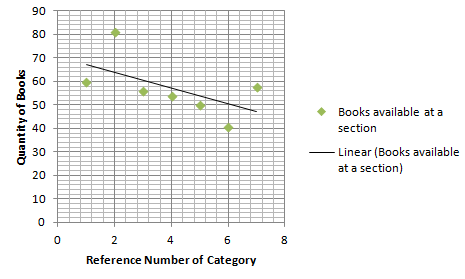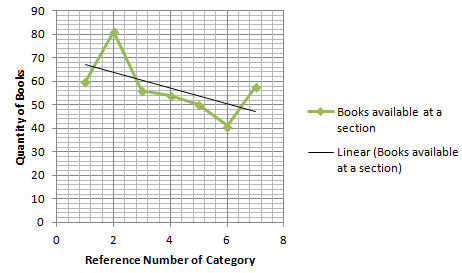My Personal Notes arrow_drop_up